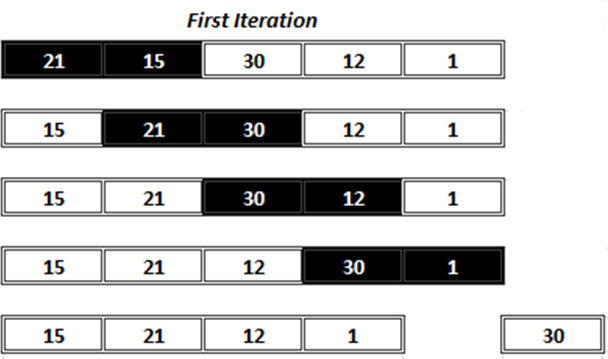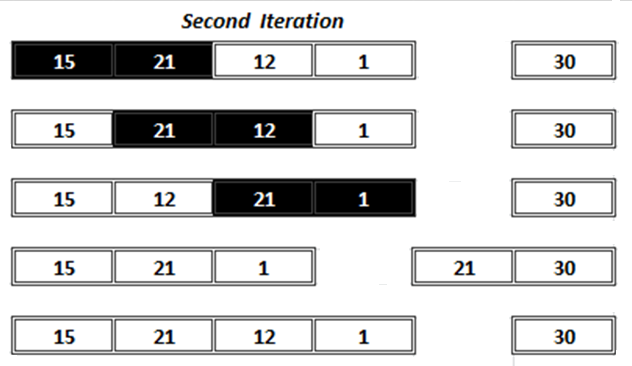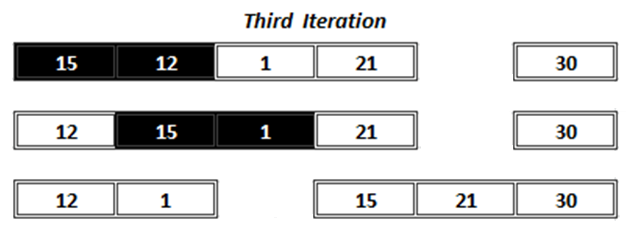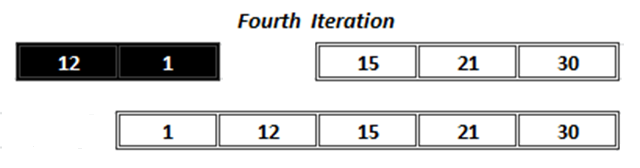• Hello there. In my last post I you learnt about searching techniques in which I explained two most common searching techniques i.e. linear search and binary search.
• In this post again I am going to discuss another most common operation that we often require to perform on the data that is sorting.
• We often require to analyse the data to take some decision. Analysis requires that data should be in sorted order. So we can say that similar to searching,sorting is the another most common operation we need to perform frequently.

Sorting is a process of arranging a set of data in some order.

• There are two different methods to sort data either in ascending or in descending order.
• There are so many techniques available for sorting such as bubble sort, quick sort, merge sort, selection sort, insertion sort, quick sort etc.
• In this post I will try to explain two most common sorting techniques: Bubble sort and selection sort.

### Bubble Sort

• Bubble sort is the simplest method for sorting. In this method, to arrange elements in ascending order 0th element is compared with the 1st If it is greater than the 1st element then they are interchanged.• In the same way all elements (excluding last) are compared with their next element and interchanged if required. On completing 1st iteration the largest element is placed at the last position.
• Similarly, in the second iteration the comparisons are made till the last but one element. This time the second largest element is placed at the second last position.• After all iteration the list becomes a sorted list.

#### Bubble Sort Implementation

``````/* C Program to implement Bubble sort. */

#include "stdio.h"
#include "conio.h"

void main( )
{
int arr, n, i, temp ;
clrscr() ;
printf("Enter No.of elements (Maximum 50):");
scanf("%d",&n);

printf("Enter %d integers values...\n", n);
for (i = 0; i < n; i++)
scanf("%d",&arr[i]);
printf ( "Array before sorting:\n") ;
for ( i = 0 ; i < n ; i++ )
printf ( "%d\t", arr[i] ) ;

// bubble sort
for ( i = 0 ; i < n ; i++ )
{
for ( j = 0 ; j < n - i ; j++ )
{
if ( arr[j] > arr[j + 1] )
{
temp = arr[j] ;
arr[j] = arr[j + 1] ;
arr[j + 1] = temp ;
}
}
}
printf ( "\n Array after bubble sort:\n") ;

for ( i = 0 ; i < n ; i++ )
printf ( "%d\t", arr[i] ) ;

getch();
}
``````

### Selection Sort

• In this method, to arrange elements in ascending order 0th element is compared with all other element. If 0th element is greater than the compared element then they are interchanged.

• This way, after the first iteration the smallest element is placed at the 0th
• In the same way 1st element is compared with all other elements and after completion of second iteration, second smallest element is placed at 1st

• The same process is repeated for all other remaining elements.
• After all iteration the list becomes a sorted list.
• The above figure illustrates the process of selection sort.
``````/* C Program to implement Selection sort. */

#include "stdio.h"
#include "conio.h"

void main( )
{
int arr, n, i, temp ;

clrscr() ;

printf("Enter No.of elements (Maximum 50):");
scanf("%d",&n);

printf("Enter %d integers values...\n", n);

for (i = 0; i < n; i++)
scanf("%d",&arr[i]);

printf ( "Array before sorting:\n") ;

for ( i = 0 ; i < n ; i++ )
printf ( "%d\t", arr[i] ) ;
// selection sort logic
for ( i = 0 ; i < n-1 ; i++ )
{
for ( j = i+1 ; j < n - i ; j++ )
{
if ( arr[i] > arr[j] )
{
temp = arr[i] ;
arr[i] = arr[j] ;
arr[j] = temp ;
}
}
}
printf ( "\n Array after selection sort:\n") ;

for ( i = 0 ; i < n ; i++ )
printf ( "%d\t", arr[i] ) ;

getch();
}
``````